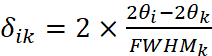## Profile calculation from peak data

Match! uses the so-called “Intermediate Lorentzian” function to calculate the profile from given Bragg peak data (2θ, intensity) and an associated FWHM (profile width) value. As the name already suggests, the profile function is something in between a Gaussian and a Lorentzian.

The intensity at each point yi is calculated from the contributions of all peaks k:The contribution of a peak k to data point i is calculated as follows:where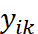is the raw (profile) intensity at point i contributed by peak k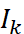is the integrated intensity of peak k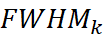is the width (“full width at half maximum”) of peak k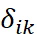is the scaled difference between the 2θ positions of point i and peak k: